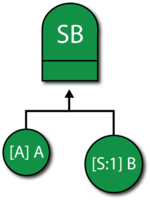# Standby Gate Example

This example also appears in the System Analysis Reference book.

### Standby GateA Standby gate behaves just like a standby container in BlockSim's RBDs. Standby containers were discussed in Time-Dependent System Reliability (Analytical) and RBDs and Analytical System Reliability. Events leading into a Standby gate have active and quiescent failure distributions, just like contained blocks. Furthermore, the gate acts as the switch, can fail and can also define the number of active blocks whose failure would cause system failure (i.e., the Active Vote Number required ). In BlockSim, no additional gates are allowed below a Standby gate.

#### Example

Consider a system with two units, A and B, in a standby configuration. Unit A is active and unit B is in a "warm" standby configuration. Furthermore, assume perfect switching (i.e., the switch cannot fail and the switch occurs instantly). Units A and B have the following failure properties:

• Block $A\,\!$ (Active):
• Failure Distribution: Weibull; $\beta = 1.5\,\!$; $\eta = 1,000\,\!$ hours.
• Block $B\,\!$ (Standby):
• Energized failure distribution: Weibull; $\beta =1.5\,\!$; $\eta = 1,000\,\!$ hours.
• Quiescent failure distribution: Weibull; $\beta =1.5\,\!$; $\eta = 2,000\,\!$ hours.

Determine the reliability of the system for 500 hours.

Solution

The fault tree diagram for this configuration is shown next and $R(t=500)=94.26%\,\!$ .